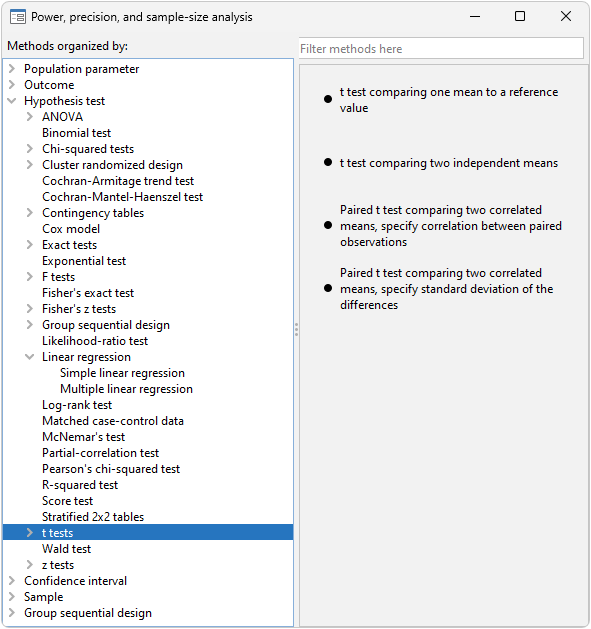Home  /  Products  /  Features  /  Power analysis for comparison of means

## Power analysis for comparison of means

### Highlights

• Classical comparisons of means
• One-sample t test
• Two-sample t test
• Paired t test
• ANOVA models
• Cluster randomized designs
• Multiple values of parameters
• Automatic and customizable tables
• Automatic and customizable graphs

Stata's power command performs various power and sample-size analysis, including classic comparisons of means.

You can compute power, sample size, and effect size. Enter any two and get the third. You can specify single values or, to compare multiple scenarios, ranges of values of study parameters. You can obtain results either in tabular form or as a graph.

### Classic mean comparisons

Stata's power provides three methods for classic comparison of means. To see the methods (and for point-and-click analysis), go to the menu Statistics -> Power, precision, and sample size and under Hypothesis test, select t tests.power onemean estimates required sample size, power, and effect size for a test comparing one mean to a reference value. You can choose between a t test or a z test and adjust for finite samples.

power twomeans estimates required sample size, power, and effect size for a test comparing two independent means. You can also compute the sample size of one group given the other. You can choose between a t test or a z test, account for unequal standard deviations, and incorporate unbalanced designs.

power pairedmeans estimates required sample size, power, and effect size for a test comparing two means from paired samples. You can choose between a t test or a z test, adjust for finite samples, and account for unequal standard deviations. To provide information about the paired design, you can specify either the correlation between the paired observations or standard deviation of the differences.

In addition, Stata's power command performs power analysis for comparisons of multiple means using ANOVA and for comparisons of means accounting for cluster randomized designs.

Say we are planning an experiment to determine whether students who prepare for the SAT exam obtain higher math scores by (1) taking classes rather than (2) studying independently. The national average math score is 520 with a standard deviation of 135. We want to see the power obtained for sample sizes of 100 through 500 when scores increase by 20, 40, 60, and 80 points or, equivalently, when average scores increase to 540, 560, 580, and 600.

We type

. power twomeans 520 (540 560 580 600), n(100 200 300 400 500) sd(135) graphWe assumed above that those studying independently would obtain the national average of 520 and that the standard deviations would be 135 for both groups. We could relax either assumption using the power command.

We might be more interested in the sample size necessary to detect the increased scores at power levels of 0.8 and 0.9. In that case, we type

. power twomeans 520 (540 560 580 600), power(0.8 0.9) sd(135) graphWe can even estimate the detectable increased scores over the range of sample sizes and powers:

. power twomeans 520, power(0.8 0.9) n(100 200 300 400 500) sd(135) graphIf we prefer to see the detectable limits as effect sizes (difference between the experimental group mean and the control group mean) rather than experimental-group test scores, we add option y(delta) to the command:

. power twomeans 520, power(0.8 0.9) n(100 200 300 400 500) sd(135) graph(y(delta))In this graph, the effect size is calculated as (experimental group mean - 520).

### Tell me more

Learn much more about these methods in the Power, Precision, and Sample-Size Reference Manual. You will find many more worked examples, extended discussion, methods and formulas, references, and more.

View all of Stata's power, precision, and sample-size features.